# seaborn.jointplot#

seaborn.jointplot(data=None, *, x=None, y=None, hue=None, kind='scatter', height=6, ratio=5, space=0.2, dropna=False, xlim=None, ylim=None, color=None, palette=None, hue_order=None, hue_norm=None, marginal_ticks=False, joint_kws=None, marginal_kws=None, **kwargs)#

Draw a plot of two variables with bivariate and univariate graphs.

This function provides a convenient interface to the `JointGrid` class, with several canned plot kinds. This is intended to be a fairly lightweight wrapper; if you need more flexibility, you should use `JointGrid` directly.

Parameters:
data`pandas.DataFrame`, `numpy.ndarray`, mapping, or sequence

Input data structure. Either a long-form collection of vectors that can be assigned to named variables or a wide-form dataset that will be internally reshaped.

x, yvectors or keys in `data`

Variables that specify positions on the x and y axes.

huevector or key in `data`

Semantic variable that is mapped to determine the color of plot elements. Semantic variable that is mapped to determine the color of plot elements.

kind{ “scatter” | “kde” | “hist” | “hex” | “reg” | “resid” }

Kind of plot to draw. See the examples for references to the underlying functions.

heightnumeric

Size of the figure (it will be square).

rationumeric

Ratio of joint axes height to marginal axes height.

spacenumeric

Space between the joint and marginal axes

dropnabool

If True, remove observations that are missing from `x` and `y`.

{x, y}limpairs of numbers

Axis limits to set before plotting.

color`matplotlib color`

Single color specification for when hue mapping is not used. Otherwise, the plot will try to hook into the matplotlib property cycle.

palettestring, list, dict, or `matplotlib.colors.Colormap`

Method for choosing the colors to use when mapping the `hue` semantic. String values are passed to `color_palette()`. List or dict values imply categorical mapping, while a colormap object implies numeric mapping.

hue_ordervector of strings

Specify the order of processing and plotting for categorical levels of the `hue` semantic.

hue_norm

Either a pair of values that set the normalization range in data units or an object that will map from data units into a [0, 1] interval. Usage implies numeric mapping.

marginal_ticksbool

If False, suppress ticks on the count/density axis of the marginal plots.

{joint, marginal}_kwsdicts

Additional keyword arguments for the plot components.

kwargs

Additional keyword arguments are passed to the function used to draw the plot on the joint Axes, superseding items in the `joint_kws` dictionary.

Returns:
`JointGrid`

An object managing multiple subplots that correspond to joint and marginal axes for plotting a bivariate relationship or distribution.

`JointGrid`

Set up a figure with joint and marginal views on bivariate data.

`PairGrid`

Set up a figure with joint and marginal views on multiple variables.

`jointplot`

Draw multiple bivariate plots with univariate marginal distributions.

Examples

In the simplest invocation, assign `x` and `y` to create a scatterplot (using `scatterplot()`) with marginal histograms (using `histplot()`):

```penguins = sns.load_dataset("penguins")
sns.jointplot(data=penguins, x="bill_length_mm", y="bill_depth_mm")
```Assigning a `hue` variable will add conditional colors to the scatterplot and draw separate density curves (using `kdeplot()`) on the marginal axes:

```sns.jointplot(data=penguins, x="bill_length_mm", y="bill_depth_mm", hue="species")
```Several different approaches to plotting are available through the `kind` parameter. Setting `kind="kde"` will draw both bivariate and univariate KDEs:

```sns.jointplot(data=penguins, x="bill_length_mm", y="bill_depth_mm", hue="species", kind="kde")
```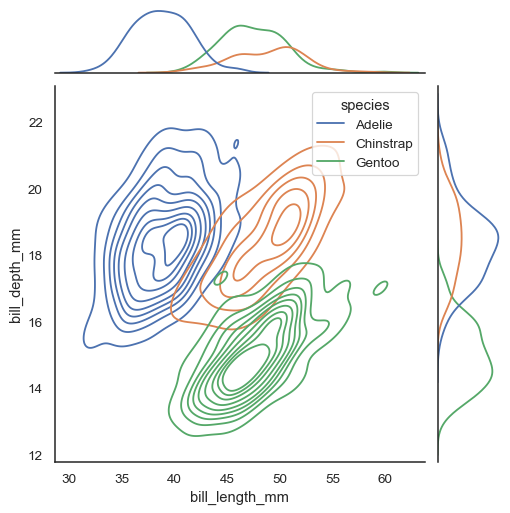Set `kind="reg"` to add a linear regression fit (using `regplot()`) and univariate KDE curves:

```sns.jointplot(data=penguins, x="bill_length_mm", y="bill_depth_mm", kind="reg")
```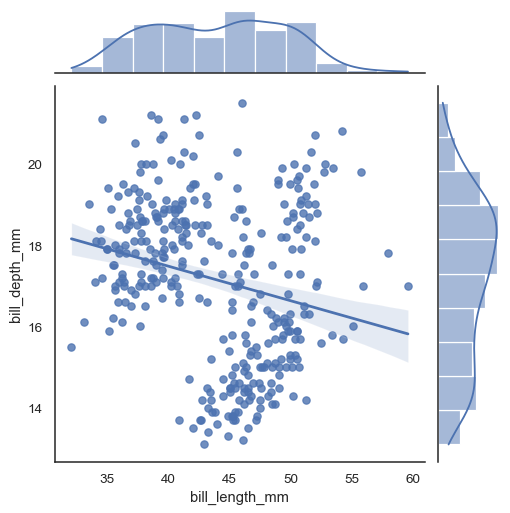There are also two options for bin-based visualization of the joint distribution. The first, with `kind="hist"`, uses `histplot()` on all of the axes:

```sns.jointplot(data=penguins, x="bill_length_mm", y="bill_depth_mm", kind="hist")
```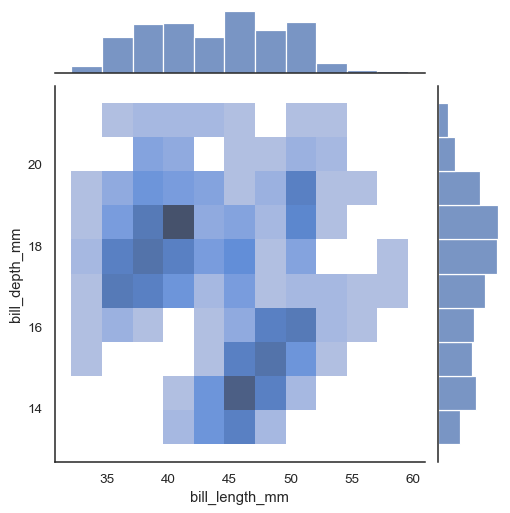Alternatively, setting `kind="hex"` will use `matplotlib.axes.Axes.hexbin()` to compute a bivariate histogram using hexagonal bins:

```sns.jointplot(data=penguins, x="bill_length_mm", y="bill_depth_mm", kind="hex")
```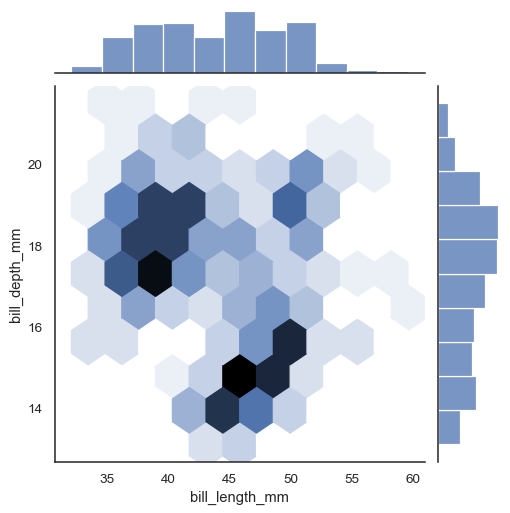Additional keyword arguments can be passed down to the underlying plots:

```sns.jointplot(
data=penguins, x="bill_length_mm", y="bill_depth_mm",
marker="+", s=100, marginal_kws=dict(bins=25, fill=False),
)
```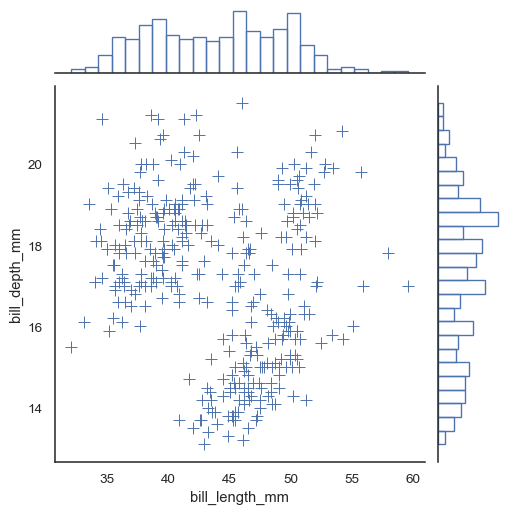Use `JointGrid` parameters to control the size and layout of the figure:

```sns.jointplot(data=penguins, x="bill_length_mm", y="bill_depth_mm", height=5, ratio=2, marginal_ticks=True)
```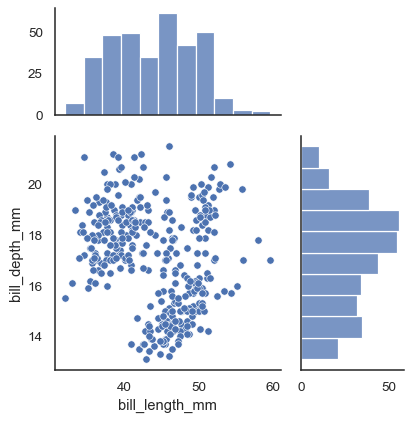To add more layers onto the plot, use the methods on the `JointGrid` object that `jointplot()` returns:

```g = sns.jointplot(data=penguins, x="bill_length_mm", y="bill_depth_mm")
g.plot_joint(sns.kdeplot, color="r", zorder=0, levels=6)
g.plot_marginals(sns.rugplot, color="r", height=-.15, clip_on=False)
```